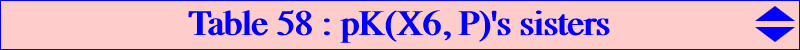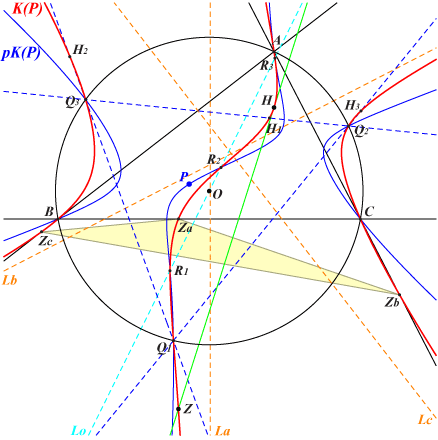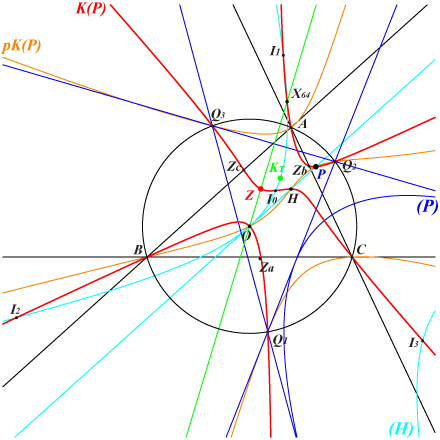Let P = u : v : w be a point and pK(P) = pK(X6, P) the isogonal pivotal cubic with pivot P. pK(P) meets the circumcircle (O) at A, B, C and three other points Q1, Q2, Q3 which are not necessarily all real nor distinct. See here for further informations. Denote by T the (possibly improper) triangle with vertices Q1, Q2, Q3. The construction of Q1, Q2, Q3 is not ruler and compass possible. It needs to intersect (O) and another conic. One solution is the homothetic under h(P, 1/2) of the polar conic of P in pK(P), a diagonal conic passing through P and the in/excenters of ABC, discarding the image of the center of the diagonal conic which is the trilinear pole of the line through X(6) and the barycentric square of P. For example, with P = X(2), X(3), X(6), T is the Thomson triangle, CircumNormal triangle, Grebe triangle respectively. These are proper and acute triangles for any reference triangle ABC. With P = X(1), T is the circumcevian triangle of X(1). Obviously O is the circumcenter of T and P is its orthocenter. The polar circle of T is that with center P and radius : -((c^2 u v+b^2 u w+a^2 v w)/(2 (u+v+w)^2)) hence T is acutangle for P inside (O). Recall that T is a proper triangle if and only if P is (strictly) inside aH3, the anticomplement of the Steiner deltoid, which is the case when P is (strictly) inside (O). Let ΦP be the isogonal conjugation with respect to T. ΦP swaps the vertices of ABC and the infinite points of its altitudes, also O and P. ΦP obviously fixes the in/excenters of T. It follows that an isogonal pivotal cubic with respect to T which is also a circumcubic in ABC must have its pivot at H and therefore passes through the vertices of the cevian triangle H1H2H3 of H in Q1Q2Q3. Hence, any pK(P) = pK(X6, P) is associated with a unique cubic K(P) we call the sister of pK(P). In other words, K(P) is the pivotal cubic with pivot H which is invariant under ΦP, the isogonal conjugation with respect to T. K(P) contains seven fixed points namely A, B, C, H and the infinite points of the altitudes of ABC hence these cubics belong to a same net of cubics with asymptotes La, Lb, Lc perpendicular to the sidelines of ABC or parallel to those of the Darboux cubic K004.K(P) also contains : • Q1, Q2, Q3, • the in/excenters of T (not represented), • the ΦP-image Z of H which is the isopivot hence the common tangential of H, Q1, Q2, Q3 in K(P). This point has first barycentric coordinate : a^2 (a^4 u-a^2 b^2 u-a^2 c^2 u+a^4 v-a^2 b^2 v+b^2 c^2 v-c^4 v+a^4 w-b^4 w-a^2 c^2 w+b^2 c^2 w), and can be construed as the Ceva conjugate of X(3) x tcP and X(6). See ETC pairs {P, Z} and {Z, P} at the bottom of this page. The mapping P –> Z is affine with fixed points X(110), X(2574), X(2575). See the related cubic K839. It can easily be verified that K(P) contains the vertices Za, Zb, Zc of the pedal triangle of Z which makes already 21 clearly identified points on K(P).K(P) meets pK(P) at six points on (O) and three other (not always all real) collinear points R1, R2, R3 on a line Lo passing through O. K(P) meets K004 at three points at infinity, A, B, C, H hence two other (not always real) points S1, S2 which lie on a line passing through Z. *** The orthic line of K(P) is the Euler line for every P. This means that the polar conic of any point M on the Euler line with respect to any cubic K(P) is a rectangular hyperbola. This is in particular the case of H (which lies on every K(P)). The orthic line of the hessian H(P) of K(P) passes through H. It coincides with the Euler line if and only if P lies on a rectangular hyperbola passing through X(4), X(20), X(2574), X(2575), X(7691). Further details and developments are available in the paper Bi-isogonal and Tri-isogonal Pivotal Cubics.Special cubics K(P) Since the infinite points are those of the altitudes of ABC, K(P) cannot be circular nor equilateral unless its decomposes. • K(P) passes through P if and only if either : – P lies on the line at infinity in which case it must decompose since it has already three known points at infinity. This is excluded in the sequel. – P lies on (O) in which case K(P) meets (O) again at two points lying on the parallel at O to the Simson line of P. See K839 for instance. – P lies on the Euler. See below. • K(P) is a K0 (and at the same time a K+) if and only if P lies on the line passing through X20, X185, X193, X194, X511, X3164, X5889 etc. When P = X20, K(P) is the Darboux cubic (which is therefore its own sister) and when P = X5889, K(P) splits into the altitudes of ABC. These are the only cases for which K(P) is a K++. The asymptotes concur at X on the Euler line and Z lies on the line X(4), X(6). See K840 for instance. • K(P) is a psK (or a pK) if and only if P lies on a central cubic with center H, passing through X20, X3146. See K841 for instance and obviously also K004. • K(P) is a nK (or a nK0) if and only if P lies on the line at infinity (see above) or on a conic with center H passing through X110, X1897. In this latter case, Z lies on (O) hence Za, Zb, Zc are collinear on the Simson line of Z. The pole of the nK is the barycentric product H x Z and lies on the circum-conic with perspector X(25), passing through X(112), X(648), X(1783). The root is the tripole of the Simson line of Z hence it lies on the Simson cubic K010. K(P) meets (O) at Z and two other points on the Steiner line of Z. See K839 and K842 for instance. There are two nK0s of this type obtained when Z is an intersection of (O) and the line X(4), X(6). These points and the corresponding points P are complicated and unlisted in ETC.Selected examples The table below shows a selection of cubics pK(P) and their sisters K(P). This table was built with contributions by Peter Moses. Recall that K(P) is always a circumcubic passing through H and having three real asymptotes perpendicular to the sidelines of ABC.P pK(P) K(P) Z Xi on K(P) for i = remarks X1 bisectors K838 X14529 4, 14529 T is the circumcevian triangle of X1, TCCT 6.22 X2 K002 K615 X154 2, 3, 4, 64, 154, 3424, 5373 T is the Thomson triangle, K(P) is spK(X20, X376) X3 K003 K405 X6759 3, 4, 64, 1676, 1677, 6759 T is the CircumNormal triangle, K(P) is spK(X20, X550) X4 K006 K376 X3 3, 4, 64, 6523 K(P) is psK(X25, X2, X3) and spK(X20, X3) X5 K005 K848 X10282 3, 4, 5, 64, 10282 K(P) is spK(X20, X548) X6 K102 K642 X206 4, 206, 1676, 1677 T is the Grebe triangle X20 K004 K004 X1498 see the page K004 T is the circumcevian triangle of X3, TCCT 6.12 X27 K109 X2328 3, 4, 27, 64, 2328 X99 K035 4, 99, 1379, 1380 X110 K316 K839 X110 4, 110, 1113, 1114 K(P) is nK(X112, X2407, X4) X185 K840 X2883 4, 2883 K(P) is a K0+ X193 X6 4, 6 K(P) is a K0+ X194 4 K(P) is a K0+ X382 K846 X3357 3, 4, 64, 382, 3357 K(P) is spK(X20, X5) X476 K130 4, 74, 110, 476 X631 K832 X14530 3, 4, 64, 631, 3338, 3527, 14530 X1351 K843 X182 4, 182, 1344, 1345, 1676, 1677 X1897 K842 X109 4, 109 K(P) is nK(X6 x X653, X2406, X4) X3146 K841 X64 3, 4, 64, 3146 K(P) is psK(X64, X253, X4) and spK(X20, X4) X3149 K844 3, 4, 56, 64, 945, 3149, 5687, 10306 X3164 4 K(P) is a K0+ X3543 K847 X10606 3, 4, 64, 10606 K(P) is spK(X20, X2) X3580 X1495 4, 74, 1495, 5000, 5001 See the related Table 55 X9965 K845 X2192 1, 4, 57, 84, 1750, 2192, 10306 X9965 = aX329 X14531 K127 4, 5, 3146 K(P) is a K0+ X14532 K820 3, 4, 64, 182, 1350Notes : Any cubic K(P) with P on the Brocard axis passes through X(1676), X(1677).Cubics K(P) with P on the Euler line When P lies on the Euler line, K(P) has a lot of specific properties. See the green cells in the table above.• P lies on K(P) as said above. • K(P) passes through X(3) and X(64). • K(P) is a member of the pencil generated by K004 and the union of the line at infinity and the Jerabek hyperbola. More precisely, K(P) is spK(X20, midpoint X20-P) as in CL055. See also Table 54, column P = X20. • K(P) is also a member of the pencil F(P) generated by pK(P) and the union of the Euler line and the circumcircle. See a study of this pencil below. • The polar conic of P is a rectangular hyperbola. • Z lies on the line X3, X64. • The sidelines of T envelope the parabola (P) which is the reflection of the Kiepert parabola about O. • The in/excenters Io, I1, I2, I3 of T, which lie on K(P), also lie on (H), the reflection of the Stammler rectangular hyperbola about O. • The Lemoine point KT of T also lie on (H).Pencils pK(P), (O) U (E), K(P) with P on the Euler line As said above, when P lies on the Euler line, K(P) belongs to the pencil F(P) generated by pK(P) and the union of the Euler line (E) and the circumcircle (O). It is clear that, for a given P, all the cubics of F(P) pass through X3, X4, P on (E) and meet (O) at the same six points. Each cubic of F(P) is spK(Q, D = midpoint P-Q) for some Q on the Euler line, meeting the line at infinity at the same points as pK(X6, Q) and passing through the foci of the inconic with center D. This pencil F(P) contains a good number of remarkable other cubics, most of them already mentioned in Table 54, although organized differently. This also allows a connection with the M-OAP points of Table 53. Indeed, F(P) always contains : • a cubic passing through the X3-OAP points, • a cubic passing through the isogonal conjugates of the X3-OAP points, • a cubic M(P) passing through the M-OAP points where M is the reflection of P about X3. These three cubics are not always all distinct. Let S be the abscissa of P in (O = X3, G = X2) i.e. OP = S OG (vectors). Consider the point Ps with abscissa (–3 – 2S) in (O, G) or, equivalently, such that X(20)Ps = –2 OP. M(P) is then spK(Ps, midpoint P-Ps) as in CL055. The following table gives a selection of these F(P), each being represented by one column. Each line also represents a pencil of cubics which share a common property.P X30 X2 X3 X20 X5 X4 X376 X381 X382 Q notes pK(X6, P) K001 K002 K003 K004 K005 K006 K243 P K(P) / ∞K004 (L)U(J) K615 K405 K004 K848 K376 K846 X20 (L1) S(P) / ∞K003 (L)U(J) K581 K003 K080 K026 K028 K309 K358 K525 X3 (L2) psK1 K447 K002 K361 K443 K849 K028 aD (L3) psK2 K446 K002 K009 K426 K026 K376 aaD X3-OAP K811 K810 K405 K814 K026 K006 K762 S (L4) X3-OAP* K854 K759 K361 K080 K006 K851 S' M X30 X376 X3 X4 X550 X20 X2 X3534 X1657 M(P) K446 K405 K443 K855 K243 Ps (L5) ∞K002 (L)U(J) K002 K047 K851 K762 X2 ∞K005 (L)U(J) K361 K566 K005 X5 ∞K006 (L)U(J) K804 K009 K443 K006 X4 F(X2) K187 K002 K706 K358 s(X2) (L6) F(X3) K187 K810 K003 K443 K376 K851 s(X3) F(X4) K187 K426 K006 K525 s(X4) F(X5) K187 K759 K009 K850 K005 K028 K762 K846 s(X5) (L7) F(X20) K187 K004 K855 s(X20) F(X376) K187 K615 K047 s(X376) notes (C1) (C2) (C3) (C4)Notations and comments K(P) is pK(X6, P)'s sister and S(P) is a stelloid with asymptotes parallel to those of the McCay cubic K003. S and S' are the images of D under the homotheties with center X4, ratios 4/3 and 2/3 respectively. (L)U(J) is the union of the line at infinity and the Jerabek hyperbola. In the line ∞Knnn, all the cubics pass through the infinite points of Knnn. In the line F(Xi), all the cubics pass through the foci of the inconic with center Xi and s(Xi) is the reflection of P about Xi. *** Notes • (L1) : the pencil of Euler's sisters also contains K820, K841, K844, K847. This column P = X20 in Table 54. • (L2) : the pencil of McCay stelloids also contains K665, K852. • (L3) : the pencil of psK1s also contains K813, K841. • (L4) : the pencil of X3-OAP cubics also contains K812, K813. • (L5) : the pencil of M-OAP cubics also contains the stelloid K665 (M = X5), K855 (M = X20). • (L6) : the pencil of cubics passing through the foci of the Steiner inellipse also contains K812, K847. • (L7) : the pencil of cubics passing through the foci of the MacBeath inconic have the same tangents at X3, X4 passing through X74 except when P = X3, X4 since the corresponding cubics K009, K028 are nodal.   • (C1) : all the cubics in this column are circular and pass through X(30), X(74). • (C2) : all the cubics in this column pass through X(2) and the vertices of the Thomson triangle. • (C3) : all the cubics in this column are CircumNormal cubics. Additional cubic : K664. • (C4) : all the cubics in this column are central with center O.ETC pairs {P, Z} and {Z, P} Here are lists of pairs {P, Z} and {Z, P} computed by Peter Moses, updated 2016-11-02.Pairs {P, Z} {2,154}, {3,6759}, {4,3}, {5,10282}, {6,206}, {8,3556}, {20,1498}, {24,10539}, {25,9306}, {27,2328}, {29,2360}, {30,6000}, {51,10192}, {52,5}, {63,10537}, {68,26}, {69,159}, {74,9934}, {92,3185}, {110,110}, {113,1511}, {144,3197}, {145,221}, {146,2935}, {155,1147}, {185,2883}, {186,10540}, {193,6}, {195,10274}, {264,160}, {317,157}, {324,418}, {382,3357}, {385,1971}, {394,1660}, {428,3819}, {511,1503}, {512,3566}, {514,8676}, {517,6001}, {518,3827}, {519,2390}, {520,523}, {521,513}, {523,924}, {524,2393}, {525,512}, {539,1154}, {542,2781}, {648,1576}, {651,692}, {732,3852}, {850,669}, {895,1177}, {912,517}, {916,516}, {952,2818}, {1112,5972}, {1147,156}, {1351,182}, {1370,1619}, {1824,4640}, {1829,960}, {1843,141}, {1897,109}, {1902,9943}, {1986,113}, {1993,184}, {2451,9426}, {2501,8651}, {2574,2574}, {2575,2575}, {2771,2778}, {2850,8674}, {2888,2917}, {2987,1976}, {2996,5023}, {3060,2}, {3146,64}, {3187,31}, {3193,1437}, {3218,10535}, {3219,10536}, {3448,10117}, {3543,10606}, {3564,511}, {3569,5027}, {3575,5907}, {3580,1495}, {3732,101}, {3868,1}, {4240,5502}, {4391,667}, {5095,6593}, {5392,3135}, {5446,140}, {5663,2777}, {5889,4}, {5905,55}, {5921,1350}, {5942,198}, {6152,1209}, {6193,155}, {6241,5878}, {6242,6288}, {6353,8780}, {6368,1510}, {6392,3053}, {6403,1352}, {6515,25}, {7253,3733}, {7576,5891}, {7703,7712}, {7722,7728}, {7754,32}, {7762,39}, {8057,520}, {8673,525}, {8677,900}, {8681,524}, {8878,10329}, {9007,8675}, {9028,674}, {9031,9002}, {9033,526}, {9051,9001}, {9190,9192}, {9308,577}, {9517,690}, {9927,1658}, {9965,2192}, {9979,351}, {10015,1960}, {10340,3499}.   Pairs {Z, P} {1,3868}, {2,3060}, {3,4}, {4,5889}, {5,52}, {6,193}, {25,6515}, {26,68}, {31,3187}, {32,7754}, {39,7762}, {55,5905}, {64,3146}, {101,3732}, {109,1897}, {110,110}, {113,1986}, {140,5446}, {141,1843}, {154,2}, {155,6193}, {156,1147}, {157,317}, {159,69}, {160,264}, {182,1351}, {184,1993}, {198,5942}, {206,6}, {221,145}, {351,9979}, {418,324}, {511,3564}, {512,525}, {513,521}, {516,916}, {517,912}, {520,8057}, {523,520}, {524,8681}, {525,8673}, {526,9033}, {577,9308}, {667,4391}, {669,850}, {674,9028}, {690,9517}, {692,651}, {900,8677}, {924,523}, {960,1829}, {1147,155}, {1154,539}, {1177,895}, {1209,6152}, {1350,5921}, {1352,6403}, {1437,3193}, {1495,3580}, {1498,20}, {1503,511}, {1510,6368}, {1511,113}, {1576,648}, {1619,1370}, {1658,9927}, {1660,394}, {1960,10015}, {1971,385}, {1976,2987}, {2192,9965}, {2328,27}, {2360,29}, {2390,519}, {2393,524}, {2574,2574}, {2575,2575}, {2777,5663}, {2778,2771}, {2781,542}, {2818,952}, {2883,185}, {2917,2888}, {2935,146}, {3053,6392}, {3135,5392}, {3185,92}, {3197,144}, {3357,382}, {3499,10340}, {3556,8}, {3566,512}, {3733,7253}, {3819,428}, {3827,518}, {3852,732}, {4640,1824}, {5023,2996}, {5027,3569}, {5502,4240}, {5878,6241}, {5891,7576}, {5907,3575}, {5972,1112}, {6000,30}, {6001,517}, {6288,6242}, {6593,5095}, {6759,3}, {7712,7703}, {7728,7722}, {8651,2501}, {8674,2850}, {8675,9007}, {8676,514}, {8780,6353}, {9001,9051}, {9002,9031}, {9192,9190}, {9306,25}, {9426,2451}, {9934,74}, {9943,1902}, {10117,3448}, {10192,51}, {10274,195}, {10282,5}, {10329,8878}, {10535,3218}, {10536,3219}, {10537,63}, {10539,24}, {10540,186}, {10606,3543}.